This article was completed in about 1980 and never published. Anyone interested in publishing it, or continuing the line of research, should get in touch with me.

### ABSTRACT

A world is divided among revenue maximizing nations, constrained by competition among themselves via costless migration. In migration equilibrium high taxes in a country are balanced by the attractiveness of the resulting low population density. It is shown that in revenue maximizing Cournot equilibrium (of tax rates), the country with larger population (but lower density) has higher taxes. The relation is tested using metropolitan areas as worlds, cities as nations. The results confirm the prediction, even after any overall dependency of taxes on population has been removed by regressing taxes on population for all cities and taking the residuals.

### A Competitive Model of Exploitive Taxation

In a previous paper (Friedman 1977), I attempted to explain the size and shape of nations on the assumption that nations were net revenue maximizers competing for land, with. the "optimum" configuration of borders depending on the characteristics of the tax base available to be exploited. One of the situations considered was that in which the income from labor (as opposed to rent on land or the "rent" from the possession of trade routes) was the major tax base available, with tax levels limited by competition among nations for taxpayers. The purpose of this paper is to explicitly model such a situation, to derive conclusions, and to test them. Boundaries will be taken as given; the purpose of the analysis is to predict, for a given division of a "world" among "nations", the resulting pattern of tax levels and distribution of population.

We begin with a world divided into nations. Within this world migration is costless. Each nation contains a certain amount of a homogeneous resource called "land"; the amount may.be different for different nations. This amount is called the "area" of the nation. It may be thought of as a measure of the geographical area of the nation weighted for quality, but need not be; its crucial characteristics are that it is immobile and capable of being crowded. There is an attractiveness function which measures the attractiveness of living in a nation as a function of population density-the population of the nation divided by its area. This function is assumed to decrease with increasing density, at least over the ranges of density relevant to our analysis. In order to derive certain results a further restriction on the attractiveness function is required; this is a marginal condition somewhat weaker than the requirement that equal percentage increases in population density result in increasingly large decreases in attractiveness as density increases.

The world has a fixed population of identical individuals, divided, at any instant, among the various nations. The governments of these nations are net revenue maximizers, and engage in pure exploitive taxation-taxation designed to enrich the governments at the expense of the population. This taxation can be thought of as an annual head tax, measured in the same units as the attractiveness function.

For any given structure of taxes among the nations, since migration is costless, the population will adjust itself so that the net advantage of living in each nation-the attractiveness function for the population density of that nation minus its tax level-is the same. The nation with higher taxes will have a lower population density, hence a higher value of the attractiveness function. Each nation thus faces an implicit supply function for taxpayers-given the tax rates that other nations charge, its population will depend on its tax rate. The exact form of this supply function depends on the areas of the nations, the tax rates of the other nations, and the form of the attractiveness function.

The nations are assumed to be in a revenue maximizing Cournot equilibrium. Each sets its tax rate at that level which maximizes revenue (population times taxes) given the tax rates of the other nations. The mathematics of the model are worked out in Appendix I. It is shown that in equilibrium if one nation has a larger area than another it will have a higher level of taxes, a lower population density, but a higher population. It is this prediction-in particular the connection between higher population and higher taxes-which I will test.

So far I have only discussed exploitive taxation. Suppose a nation finds that there is some service it can provide to its inhabitants which they value at more than its cost. Providing this service (and raising taxes accordingly) makes the nation on net more attractive; part or all of this gain can then be extracted by additional exploitive taxes. Hence it pays the nation to provide all such services; the taxes that pay for them I will call "non-exploitive." Provided that the. opportunities for providing services funded by non-exploitive taxation do not vary with population, area, or population density over the relevant range, my conclusions are unaffected; each nation will have, in addition to its revenue maximizing level of exploitive taxation, the same level of non-exploitive taxation. I will assume that this condition is met at least sufficiently well to leave my conclusions about the relation of exploitive taxation to population unaffected .

#### Testing the Model

In constructing a test for my model, several problems arise. The first is that area, in the sense of the model, is not measurable-even if it is thought of as corresponding to land area, it must be weighted by the quality of the land; Holland may, for our purposes, be bigger than Norway or Libya. In addition, area in this sense may include characteristics unrelated to physical land area, such as the ability of the atmosphere in a particular place to carry off pollution, an ability which affects how high a population is necessary to decrease the attractiveness of the area by a given amount. One solution to this difficulty would be to test the theory on a world, or a set of worlds, within which relevant characteristics were very uniform, so that area in the sense of the model would be closely related to geographical area. I prefer instead to take advantage of the fact that one of the predicted relations-that between the total population of a nation and its level of taxes-can be tested without requiring any measure of the area of the nation.

A second difficulty in testing the model is that individuals were assumed identical. Individuals come into the structure of the model in two ways-that is to say, there are two ways in which the analysis would change if we considered three people to be an "individual," or half a person. First, the individual is the unit for receiving the benefit expressed in the attractiveness function. Three individuals receive three times as much attractiveness from a given population density as compared to one individual. They are therefore willing (given the alternative of emigration to a denser, hence less attractive, country) to pay three times the taxes. Second, the individual is the unit determining population density; three individuals moving to a country increase its population density by three times as much as one individual.

It is not obvious whether an individual in the literal sense of a person is the best measurable unit to use for these two purposes. If we assume that the (dollar) attractiveness which a person receives from a given population density is proportional to his income and that the crowding effects which he imposes (by, for instance, buying and occupying land) are also proportional to his income, then the natural unit to use -the unit that ought to correspond to the "individual" of the model -is not a person but a dollar of income earning ability. On that assumption, instead of comparing populations and per capita tax levels, I ought, in testing the model, to compare the total income earned within each nation and the per dollar tax level-the total taxes divided by the total income. In the tests described below, I apply the model both on the assumption that the "individual" of the model corresponds to a person, and on the assumption that it corresponds to a dollar of income earning ability, and test it both ways.

The next problem in testing the model is finding a world, or better yet a set of worlds, to which the model may reasonably be applied, and for which data exist. The problem which inspired the model was that of a population with a common language and culture, divided among a number of nations; I argued (Friedman 1977) that the larger the number of nations, the lower, because of competition, would be the equilibrium tax rate. Unfortunately that particular application involves substantial problems, both in defining the relevant worlds and in comparability of measured tax levels; in addition in some cases, such as the two Germanies, the assumption of costless migration is somewhat implausible. I have accordingly not chosen to test the model in that application here. A second possibility would be to take as the world the U.S., and as nations the states. In this case tax levels are easily compared (aside from the question of whether local taxes as well as state taxes should be included) and it is arguable that migration costs are low enough to make the model applicable. The difficulty here is that although as a matter of strict logic the ordering predictions of the model apply however large the number of nations in a world, the size of the differences in the equilibrium level of exploitive taxes between nations of different sizes becomes small if the number of nations is large and the fraction of the population held by the largest is a small part of the total; this is shown in Appendix I (see equation 13 for an example with a particular attractiveness function). The reason is that the expression for the tax level in one country involves a sum over all countries except that one; where the number of countries is large and the largest a small part of the whole, the sum is almost independent of which country is omitted. In the simple case shown in equation 13 the result is that the predicted level of exploitive taxation for California is only about ten percent higher than that for Alaska. An effect that small, even if it exists, is unlikely to be detectable, given the existence of substantial error terms involving other determinants of either exploitive or non-exploitive taxes.

A third possibility for testing the model is to take as my worlds metropolitan areas, and as my nations the cities within those areas. In this case the assumption of zero migration costs is even more reasonable than with states. Metropolitan areas generally have a small number of large cities, which among them contain most of the population of an area. Furthermore, the number of metropolitan areas is large enough to provide a reasonable sample for statistical tests. And tax levels among cities, especially among cities within the same state, can be directly compared, without any problem of exchange rates arising as in the international case, and without any serious problem in defining what is or is not a tax. I accordingly chose to test my model by seeing to what degree the ordering of cities within metropolitan areas by taxes was predicted by the ordering by population.

One problem arises in attempting any statistical test of my model. The prediction of the model concerns the relation between two orderings- the ordering of cities by population (or income) and by per capita taxes (or per dollar taxes). The conformity of the data to this prediction may be directly tested by ordering statistics. In doing so, one ignores the size of differences and considers only their sign. Intuitively, it seems obvious that a situation in which the city whose population is one percent higher has a tax level one percent lower is a less serious contradiction to my prediction than a situation where the figures are both fifty percent; ordering statistics treat the two cases as identical. One is thus in some sense throwing away data. The problem is that in order to use that data- by running regressions on populations and tax levels instead of tests on their order-one requires an explicit predicted relation between the variables. In the model that particular relation depends.on the particular form of the attractiveness function-which is not known. Hence any particular regression runs the risk of failing to support the theory, not because it is wrong, but because the form of relation tested by the regression is not the form implied by the theory for the particular (unknown) attractiveness function that actually exists.

Because of the existence of these problems, I have used both ordering statistics and regressions to test the predictions of the theory, and report the results of both.

One final problem remains. Measured taxation is the sum of exploitive and non-exploitive taxation. I have assumed that variation in non-exploitive taxation with population, density, and area is not sufficient to alter the prediction of the model with regard to exploitive taxation. It is nonetheless possible that it may be large enough to produce correlations between measured taxation and population which have nothing to do with the predicted variation in exploitive taxation. The way in which this problem was dealt with will be described below.

#### The Tests

My data set consisted of all of the metropolitan areas in the list of SMSA's in the 1979 Statistical Abstract, such that the name of the SMSA contained the names of at least two cities which were listed cities with population of 25,000 or more given in the 1972 County and City Data Book. For each city I took the population, total taxes, and per capita money income as given in the latter source. The result was a collection of twenty-nine metropolitan areas containing two cities each, plus sixteen more containing three cities each. The list of areas and cities is given in Appendix II. Of these, two of the two-city areas and one of the three-city areas contained cities located in two different states. Reported results include all of the metropolitan areas, including the three interstate ones, unless otherwise stated.

My first test was to compare the actual ordering within each metropolitan area of cities by per capita taxes with the ordering by population. A similar test was done using per dollar taxes (per capita taxes/per capita income) and income (per capita income times population). The results were tested for significance using the null hypothesis that the orderings were unrelated. In calculating the significance of the results for the ordering of triples, I used as my measure of goodness the sum of squares of deviations between predicted and actual order. For the pairs, the criterion was simply the number of pairs whose order was predicted correctly. The probability of doing as well or better was calculated separately for the pairs and for the triples (by exact calculation) and the two significance levels were then combined by the conventional rule of adding chi squares and degrees of freedom to yield a combined significance level. The results and their significance are reported on lines l and 3 of Table 1. Both the income and the population tests yield a significance of better than .01.

At this point, the following objection may be made to regarding these results as serious evidence for the model. There exist numerous reasons why one might expect that larger cities would on average have higher taxes-diseconomies of scale, increased loss of control by the taxpayers due to the public good nature of voting, et multa cetera. If larger cities have on average higher taxes, then within any metropolitan area per capita taxes will tend to increase with population, hence my results can be expected without my theory. In statistical terms, this objection suggests that the null hypothesis of no relation is an inappropriate one.

I deal with this problem, within the context of ordering statistics, as follows. Assume that there is same general tendency for taxes to increase with population; within my model one may think of this as a characteristic of the non-exploitive part of the tax. There is no obvious reason why such a tendency should depend on metropolitan area as well as population. I therefore take my entire sample of 106 cities and regress per capita taxes on population. Insofar as there is, in addition to this general tendency, a further tendency (in the exploitive part of the tax) for per capita taxes to increase with population within metropolitan areas, the result will be to overestimate the regression coefficient so far as the general tendency is concerned. If I then take the residuals of that regression, I will have subtracted out all of the general tendency for taxes to increase with population, as well as some fraction (reflecting the overestimate of the coefficient) of the tendency for them to do so within metropolitan areas. If I then do ordering statistics on the residuals, in precisely the same way as I previously did them on the per capita taxes themselves, the results should if anything underestimate the degree to which taxes within a metropolitan area vary with population in addition to any general variation that applies both within and across metropolitan areas. I have done this using as before, both income and population; the results are reported on lines 2 and 4 of table I. In the case of the income calculations, the result is unaffected by the change; in the case of population, the significance is somewhat weakened. Table 2 shows the results of the regression of per capita taxes on population (line 1) and per dollar taxation on income (line 3); it is worth noting that the t values are small.

So far I have reported the results of ordering tests. In addition I ran a regression of per capita taxes on average population in the metropolitan area and the difference between the population of the city and the average population of its metropolitan area. If the connection between per capita taxes and population applies uniformly across metropolitan areas, the two coefficients (of average population in the area and of the deviation from the average for the particular city) should be equal. If, as my model suggests, there is a tendency for the larger city within a metropolitan area to have higher taxes which exists in addition to any general tendency for larger cities to have higher taxes, then the second coefficient should be larger than the first. The results of that regression and of a similar regression using per dollar taxes and income are given in lines 2 and 4 of table 2. The difference between the two coefficients has the predicted sign, but is insignificant; in each case t, the ratio of the difference to its standard deviation estimate, is less than one.

In discussing my selection of data, I mentioned that for three of the metropolitan areas the cities considered were in two different states. Such a situation presents a difficulty for the model, since migration decisions would presumably take into account differences in state taxes as well as in city taxes. I therefore redid the calculations eliminating the three metropolitan areas in question. The result was in every case to improve the results, but the improvement was not sufficient to make the differences in coefficients reported in table II significant.

#### Conclusion

The results of my tests appear to me to strongly confirm the prediction of the model. Even after eliminating the general tendency for taxes to increase with size (measured by population or income) in a fashion which biased the results against me, the null hypothesis was still rejected at about the .01 level. The failure of the regression tests to give significant results is disappointing but not especially surprising, given that I do not know the proper form in which the relation should be tested. The signs of the differences in the fitted coefficients were in accord with my prediction, hence the results, insofar as they are evidence of anything, are (very weak) evidence in favor of the model, not against it.

There are several obvious ways in which the model could be tested further. It could be applied to county governments within metropolitan areas, thus expanding the sample. It could be applied to the governments of independently taxing subdivisions in other countries, provided their number is small-Australia is an obvious possibility. It could be applied, with more difficulties, to international comparisons within a region or language area. I hope that other workers will be sufficiently intrigued by the model and the evidence I have presented to devise and apply such tests.

#### References

Friedman, David, "A Theory of the Size and Shape of Nations", Journal of Political Economy, 85 February 1977: 59-77.

Table 1

Ordering Statistics
 Pairs Right/Wrong Triples* Significance Level 1. Population v per capita taxes 22/7 5/6/3/2 (46) .002 2. Population v the residual of per capita taxes 20/9 4/7/3/2 (48) .012 3. Income versus per dollar taxes 20/9 3/9/3/1 (44) .006 4. Income versus the residual of per dollar taxes 20/9 3/9/3/1 (44) .006

*The results for triples are in the form a/b/c/d ([[Sigma]] ([[Delta]] S)2). Here a/b/c/d are the number of triples for which, if the cities within the area are ordered by one variable (the predicted order), the order of the other variable is 123, 213 or 132, 312 or 231, 321 respectively. [[Sigma]] ([[Delta]] S)2=2b + 6c +8d is the sum of the squares of the deviation between actual and predicted order.

TABLE II

Regression Results

 Bx105 Cx105 t(C-B) t R2 1. Per capita taxes on population.* 1.95 1.05 .01 2. Per capita taxes on average population of M, A. and (population-average population of M,A.)** 1.38 2.85 .38 .012 3. Per dollar taxes on income.* .03 .2 .0004 4. Per dollar taxes on average income of M.A. and (income-average income of M.A.)** -.05 .16 .68 .005

*The fitting form is Y = A + BX

**The fitting form is Y = A + BX + CZ

### Appendix I

Consider a world containing a set of nations { i} , with populations (Ni} , Areas { Ai} , per-capita exploitive taxes { Ti} .There exists an attractiveness function Å (di), di=Ni/Ai. Å (di) is assumed continuous and twice differentiable, Å ' is continuous and <0 for di> 0.

For an equilibrium distribution of population we have: Å (di) - Ti = Å (dj) - Tj = 0 without loss of generality (1)

Since total population is fixed, we have: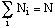(2)

Since each nation sets its tax rate to maximize its revenue subject to the tax rates of the other nations, we have: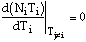(3)

We will find it useful to define two additional symbols:

riÅ '(di)/Ai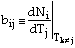where Ni(Tj) is defined implicitly by (1). Differentiating (1) with respect to Ti yields:

ribii-1=rjbji i!= j (4)

Differentiating (2) with respect to Tj yields:(5)

Since (1) involves only relative taxes, it also follows that(6)

From (3) we have: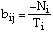(7)

Combining (4) and (5) we have:Solving for bjj gives us: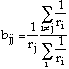(8)

Combining this with (7) we have: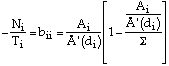(9)

hence: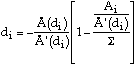(10)

where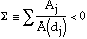From here on, without loss of generality, we will take Ai > Aj We assume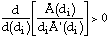We first consider the case Å (di)<Å (dj). If di >dj we have: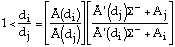(12)

where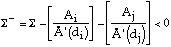It is easily shown that both brackets on the r.h.s. are positive and less than one (given that Å (di) and Å (dj) are >0, as they must be in equilibrium, since negative taxes are never optimal. Hence if Å (di) < Å (dj) then di < dj

Next we consider the case Å (di)>Å (dj)By (11) this implies that di <dj. We have now shown that:

Theorem: If Ai>Aj then di<dj and hence Ti>Tj by (1). It remains to show that Ni> Nj. Assume the contrary.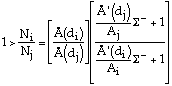Since the first part of the r.h.s. is >1, the second must be <1. It follows that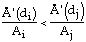Hence: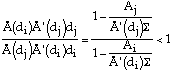Which contradicts (11) since by the Theorem, di<dj. This implies:

Improved Theorem: Ai>Aj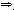di<dj, Ti> Tj, Ni> Nj.

Since for Ai=Aj it is clear that di=dj, Ti=Tj, Ni=Nj, it follows that:

Ni>NjTi>Tj. It is this prediction that I test.

Some Special Cases

i: k=2

Combining (5) and (6) one gets b11=b22, implying that: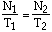ii: A simple form of Å (di).

Suppose Å (di)=B-C ln(di)

From (10) we have: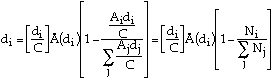(13)

This solution has two interesting characteristics in addition to simplicity. If the population of the largest nation is small compared to the total population, the level of exploitive taxation varies only slightly from one nation to another. As the number of nations increases (the distribution of sizes remaining the same) the level of exploitive taxation falls towards a non-zero limit. That these results may be expected in solutions with other forms of the attractiveness function may be seen by rewriting (9) as:If the first bracket were replaced by 1, the equation could be solved by having all nations charge a uniform tax T=-dÅ '(d), where d is the world population density. For the many nations case the bracket approaches 1, hence we would expect the value of Ti to tend towards a uniform value. This value cannot be zero unless either di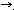0 or Å '(di)0; the latter is impossible (save for di[[infinity]] ) by continuity, since by assumption Å '(di) < 0.

### Appendix II

 Metropolitan Area Ordering Results Popn v per cap taxes pop v residual per cap income v per \$ tax and per tax residual Lawrence&endash;Haverhill Mass. - - - New Haven-W. Haven Conn. + + + New London-Norwich Conn. - - - Utica-Rome N.Y. + + + Charlotte-Gastonia N.C. + + + Fort Llauderdale-Hollywood Fla. + + + Greenville-Spartanburg S.C. + + + Huntington W.Va.-Ashland Ky.* + + + Little Rock-N. Little Rock Ark. + + + Melbourne-Titusville Fla - - - Newport News-Hampton VA + + - Raleigh-Durham N.C. - - - Tampa-St. Petersburg Fla. + + + W. Palm Beach-Boca Raton Fla. + + + Beaumont-Port Arthur Texas + - - Dallas-Fort Worth Texas + + + Appleton-Oshkosh Wis + + + Duluth Minn-Superior Wis.* - - - Hamilton-Middleton Ohio - - - Lansing-E. Lansing Mich. + + + Lorain-Elyria Ohio + + + Minneapolis-St. Paul Minn. + + + Youngstown-Warren Ohio + + + Denver-Boulder Colo. + + + Eugene-Springfield Oreg. + + + Los Angeles-Long Beach Calif + - + Salt Lake City-Ogden Utah + + + San Francisco-Oakland Calif + + + Seattle-Everett Wash. - - - Albany-Schenectady-Troy N.Y. (++) (++) (++) Allentown-Bethleham-Easton Pa. (++) (+) (++) New Brunswick-Perth Amboy-Sayreville N.J. (+) (+) (+) Patterson-Clifton-Passaic N.J. (+) (+) (+) Providence-Warwick-Pawtucket R.I. (+) (+) (+) Springfield-Chicopee-Holyoake Mass. (+) (+) (+) Greensboro-Winston-Salem-High Point N.C. (++) (++) (++) Norfolk-Virginia Beach-Portsmouth Va (+) (+) (-) Davenport Iowa-Rock Island-Moline Ill.* (--) (--) (--) Gary-Hammond-East Chicago Ind. (--) (--) (-) Anaheim-Santa Ana-Garden Grove Calif. (++) (++) (+) Oxnard-Simi Valley-Ventura Callif. (+) (+) (+) Riverside-San Bernardino-Ontario Calif. (-) (-) (-) Salinas-Seaside-Monterey Calif. (-) (-) (+) Santa Barbara-Santa maria-Lompoc Calif. (++) (++) (++) Vallejo-Fairfield-Napa Calif. (-) (-) (+)
+: Ordered according to the prediction

-: Ordered against the prediction

If ordered according to one variable, the order according to the other is

(++): 123

(+): 213 or.l32

(-): 312 or 231

(--): 321

*:The named cities are in two different states.
Back to the list of articles.